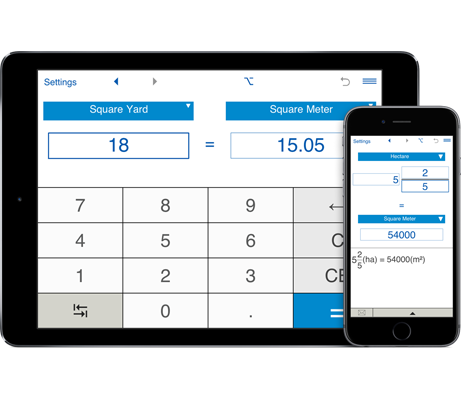# Area Converter

Area Converter is a useful application that lets you convert between US, imperial and metric area and surface units, including square inches, square feet, square yards, square miles, hectare, acre, square kilometers, square meters, square decimeters, square centimeters, and square millimeters.
You can convert between decimal and fractional values of area.

## Area Units Conversion

Area Converter helps you instantly convert between area units like square feet, square miles, hectares, acres, square meters, square kilometers and more. It is a must-have tool for students, engineers, architects and any professionals who need to regularly convert area units.
Conversion between decimal and fractional values of area. For example, you can convert fraction square feet to decimal square feet, square meters to fractional and decimal square feet, decimal square inches to fractional square inches and vice versa, fraction or decimal square inches to millimeters, etc. Area Units Converter keeps conversion history.
With the Flip button you can quickly switch between 'from' and 'to' area units.
With Area Unit Converter you can round decimal results to the required number of decimal places. For fractions, you can round results to the nearest 1/2, 1/4, 1/8, 1/16, 1/32, 1/64, 1/128, 1/256.

### What area units you can convert

This area unit converter lets you convert between different area units including square inches, square feet, square yards, square miles, hectares, acres, square kilometers, square meters, square decimeters, square centimeters, and square millimeters.

## Area and surface unit converter Features

You can convert between decimal and fractional values of area units:
• Fast conversion between US, imperial and metric area units
you can instantly convert area in metric units to inches, feet, yards, acres and miles in a decimal or fraction form and vice versa. Results are shown as decimals and fractions. For example, you can easily calculate that 12(km²) = 4.6332259(sq mi) ≈ 4.63(sq mi) in decimals and 12(km²) ≈ 4 5/8(sq mi) in fractions
38(ha) = 93.90004496(ac) ≈ 93.90(ac) in decimals and 38(ha) ≈ 93 7/8(ac) in fractions
380(mm²) = 0.58900118(sq in) ≈ 0.59(sq in) in decimals and 380(mm²) ≈ 5/8(sq in) in fractions
or you can calculate that 10 1/2(sq in) = 67.7418(cm²) ≈ 68(cm²)
5 1/4(sq ft) = 0.48774096(m²) ≈ 0.49(m²)
10 3/8(sq yd) = 8.67482136(m²) ≈ 8.67(m²)

• you can also convert between decimal and fraction forms of US or imperial units. For example, you can find that 380(ac) = 0.59375(sq mi) ≈ 0.59(sq mi) or 380(ac) ≈ 5/8(sq mi)

• with Area Converter makes it easy to convert metric units -> metric units and imperial units -> imperial units. For example, you can calculate 120(mm²) = 1.2(cm²) or 590(ha) = 5.9(km²).

• Supported length units: square inch, square foot, square yard, square mile, hectare, acre, square kilometer, square meter, square decimeter, square centimeter, and square millimeter. For example, you can convert square inches to square millimeters (square inches to mm2), square centimeters to square inches (cm2 to sq in), square feet to square meters (ft2 to cm2), square meters to square feet (m2 to ft2), square yards to square meters (yd2 to m2), square miles to kilometers (sq mi to km2), square millimeters to square centimeters (mm2 to cm2), convert between square inches, square feet, square yards, acres and square miles and more...

• Area Converter supports rounding to any number of decimal places between 0 and 8. By default, the app displays 2 decimal places. To round results to the nearest whole number, select 0 decimal places.

• Unlike other area converter, our app lets round results to the nearest usable fraction. Area Units Converter supports rounding to the nearest 1/2, 1/4, 1/8, 1/16, 1/32, 1/64, 1/128, 1/256. By default, the app rounds fractions to the nearest 1/32. If you do not need to round fractions, just select "-" in the Settings window.

• You can send results and conversion history via email.